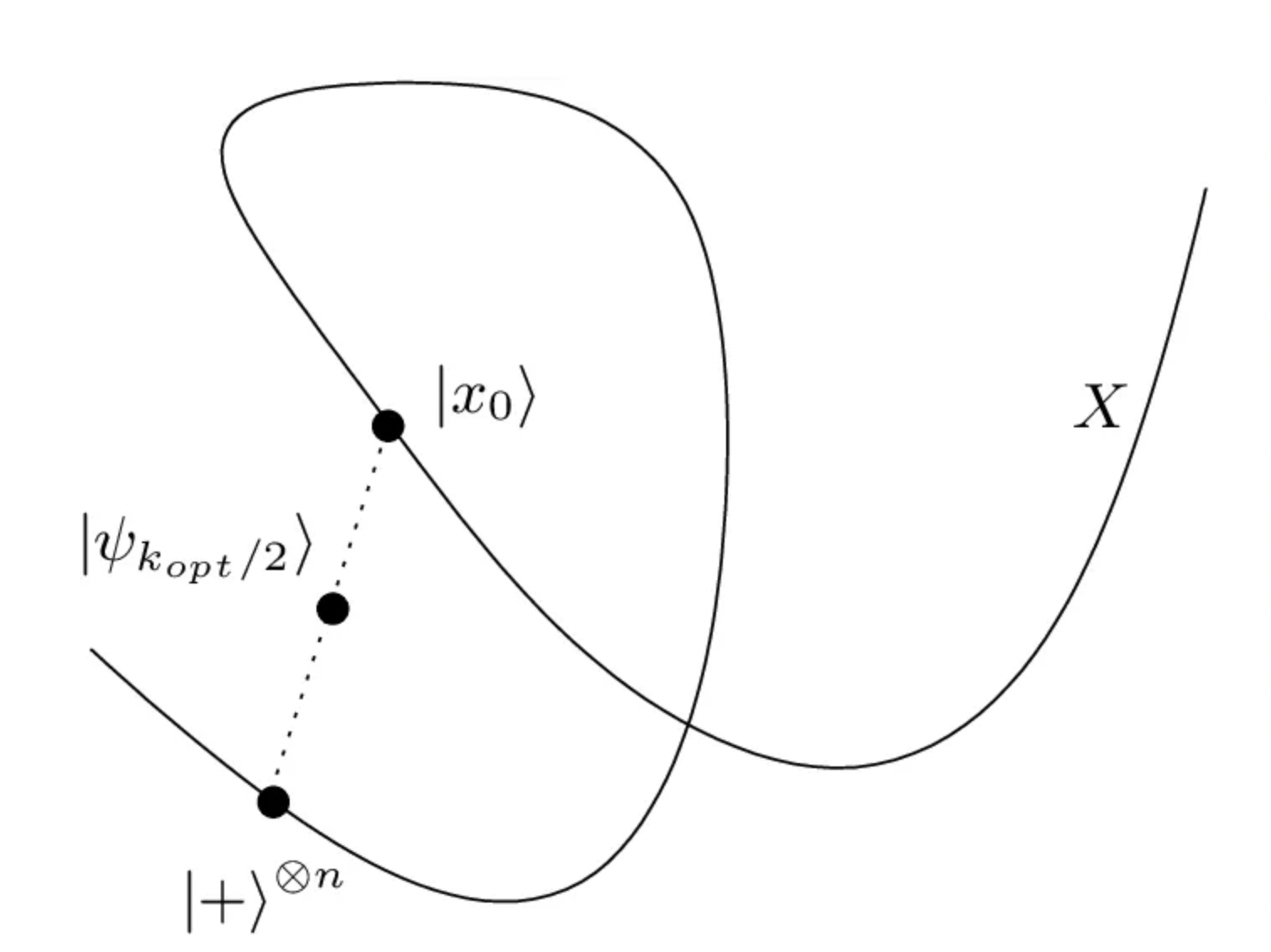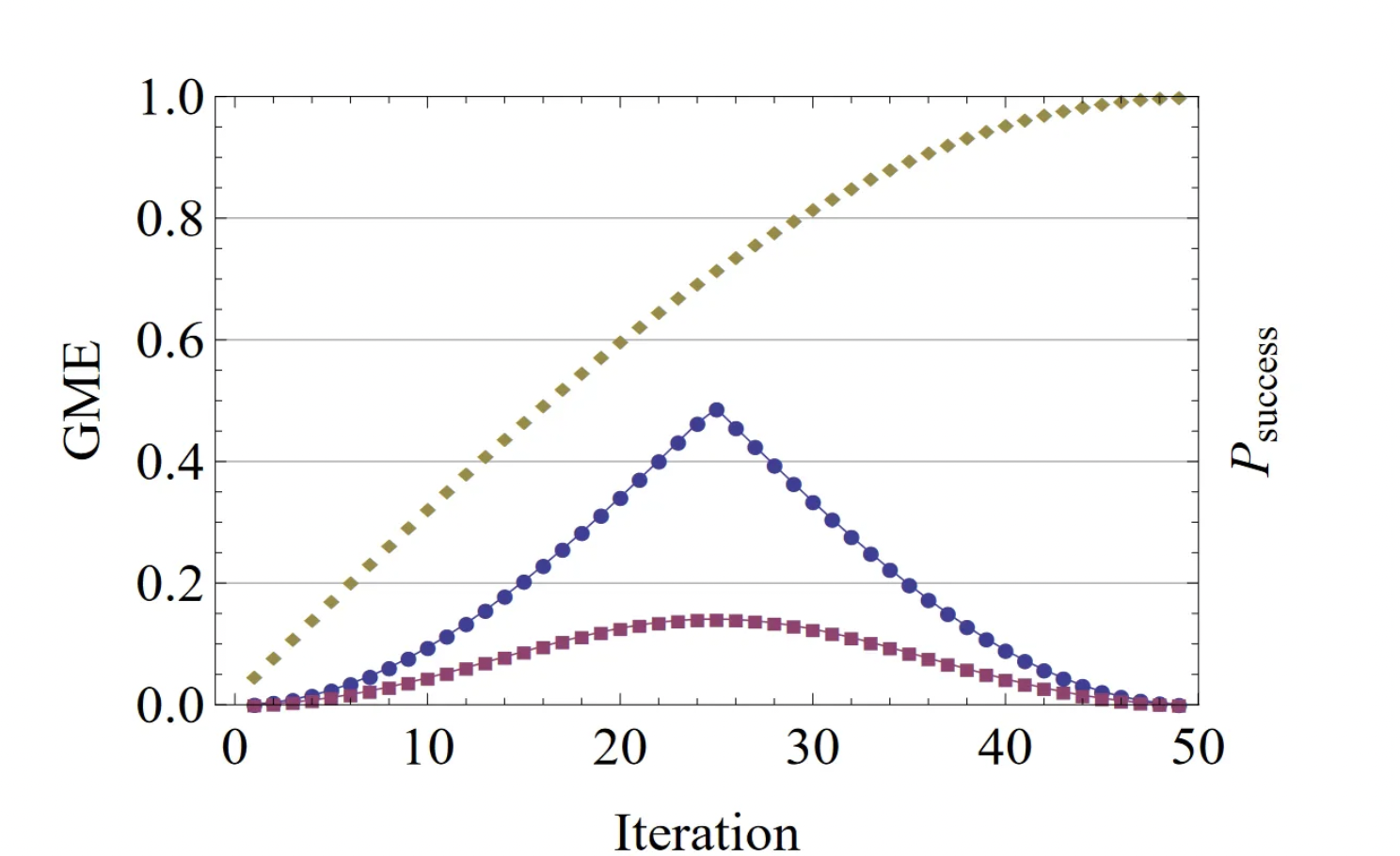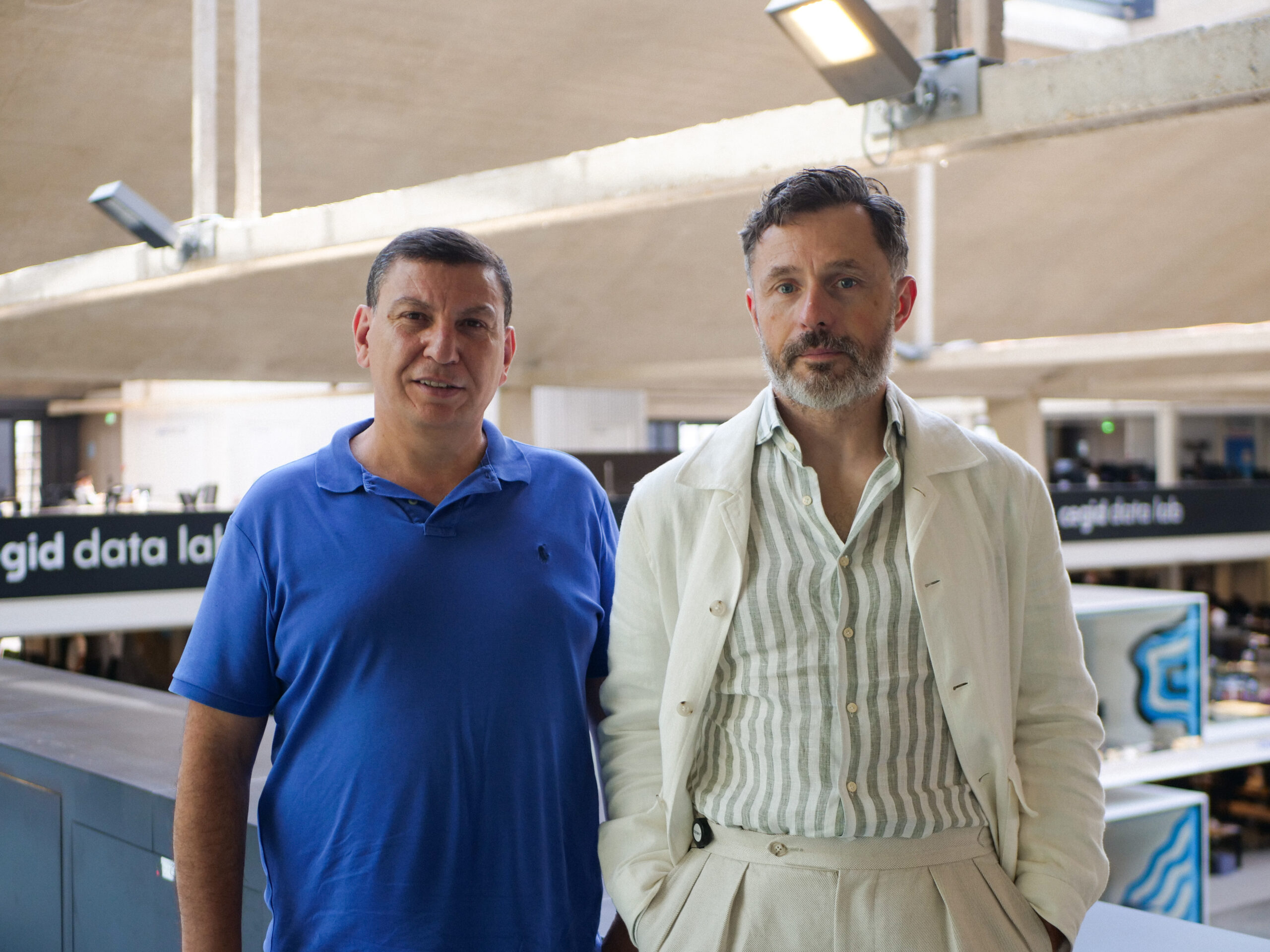# Quantum Entanglement involved in Grover’s algorithm: a state of the art

By
Hamza Jaffali
June 12, 2023
11 minutesGrover’s algorithm is a quantum search algorithm that has been widely studied since its discovery in 1996. It offers a quadratic speedup over classical search algorithms, making it an important tool in the field of quantum computing. We first introduce in a simple way how the algorithm is working. The scientific community claims that the speedup of the algorithm comes from the entanglement appearing during this algorithm. That is why, in the second part of this article, we present the works in the literature that tried to discuss the role and evolution of Quantum Entanglement in Grover’s algorithm.

## Grover’s algorithm, quick recall

Proposed by Lov Grover , this algorithm aims to solve the problem of searching for an element in an unordered database of size N. No prior information is known about the element being searched, except a function O, commonly called the Oracle, which is defined to recognize the searched element. The best classical strategy is equivalent to going through each element of the database one by one, evaluating the oracle for each element, until it is found. On average, this takes N/2 evaluations of the oracle, and in the worst case N evaluations. The complexity is therefore of order O(N) here, in terms of evaluations of the oracle. One of the great advantages of Grover’s algorithm is that it offers a complexity of order O(sqrt(N)) evaluations of the oracle.

Grover’s quantum algorithm proposes to represent the entire database as a quantum register of size N. It is assumed that N = 2for simplicity, implying that the database can be represented using a quantum system with n qubits. Each basis state corresponds to an element of the database.

There are four main steps in Grover’s algorithm: the initialization, the Oracle operator, the Diffusion operator, and the final measurement. S is the set of elements being searched when the algorithm is executed, and |S| designates the number of elements being searched. Depending on |S| and N, the Oracle and Diffusion operators could be repeated multiple times.

## Entanglement … are you here ?

The question of the role and evolution of entanglement aroused interest since the 1996’s publication. Does entanglement appear in this algorithm? If so, when and how? Does it increase through the iterations? Does it have a more complicated behavior? When do we reach a miximum of entanglement? What is the relation between entanglement and the optimal iteration? What types of entanglement are appearing through the algorithm? All these questions were studied and discussed in many research publications of the last years, and we thought interesting to regroup most of them below, providing an overview of the state of the art on that question.

## First publications on the subject

Braunstein and Pati were the first (2002) to demonstrate the presence and necessity of entanglement in Grover’s algorithm .

In 2002, Biham, Nielsen and Osborne  introduced a measure of entanglement, specifically an entanglement monotone, derived from Grover’s algorithm. Starting from a pure input state for the algorithm, the authors study the maximization Pmax of the probability of success of the algorithm, subject to the action of local unitary operations. The authors then define what is called the Groverian Measure of Entanglement.

In the same year, Forcer et al.  studied the roles of quantum superposition and entanglement in quantum computing. Their analysis is illustrated by a discussion of a classical implementation of Grover’s algorithm. The absence of multipartite entanglement implies an exponential growth in the resources required for the implementation of such quantum algorithms. The authors conclude that multipartite entanglement is the key property of quantum systems, providing remarkable power to quantum computers.

In 2003, Biham, Shapira and Shimoni analyzed the dynamics of Grover’s algorithm while initializing the algorithm with an arbitrary pure state, instead of the basis state |0…0> . The authors showed that the optimal time to perform the measurement is the same for both choices of initial states, in the case where the number of marked elements is the same. Biham et al. generalized the Groverian Measure of Entanglement to multiple marked elements. According to the authors, as long as |S| << N, the groverian measure is independent of the number of marked elements |S|.

## Dynamics and scaling of entanglement

In 2004, Orus and Latorre studied the behavior of entanglement as the system size increases in an adiabatic version of Grover’s algorithm , stating that the Von Neumann entropy remains a bounded quantity, regardless of the size of the system, even at the “critical point”. More specifically, the authors specify that the maximum entropy approaches the value 1 at a rate proportional to the square root of the system size, which is the typical scaling factor of Grover’s algorithm.

In 2005, Fang et al.  focused on studying the degree of entanglement present in a multi-qubit register during the execution of the algorithm using the density matrix formalism. The authors analyzed the variations of concurrence and Von Neumann entropy for the reduced density matrices of one or two qubits of an n-qubit register. This analysis was done as a function of the number of iterations and the number of marked elements. The calculation of concurrence showed that this measure can be related to the probability of success of the algorithm. In addition, Fang and his collaborators observed that concurrence reaches a maximum value approximately halfway through the optimal number of iterations.

In 2008, Iwai et al.  published an article on the measurement of entanglement from the perspective of the bipartition of an n-qubit system, a measure previously defined and studied from the perspective of Riemannian geometry in a work by the first author . This latter work established the distance separating maximally entangled states from separable states. In the work , the authors determine the set of maximally entangled states closest to a separable state, as one can encounter at the beginning of Grover’s algorithm. They confirm that even if the initial state and the marked elements are separable states, the algorithm generates a sequence of entangled states.

In 2012, Wen and Cao attempted to describe the behavior of multipartite entanglement in Grover’s algorithm by studying an adiabatic version of the search algorithm . The Von Neumann entropy was calculated for all possible bipartite separations of the quantum system. This led them to a new measure of entanglement, generalizing the measure introduced by Meyer and Wallach in 2002 . They demonstrate the existence and evolution of entanglement throughout the search process. Moreover, the same evolution of entanglement was observed in both the bipartite and multipartite cases (starting at zero to reach a maximum value at the critical point, then returning to zero). The symmetrical behavior of entanglement during the adiabatic evolution of the algorithm is also highlighted in this work. We can also mention the work of Pati and Braunstein in the same year .

In 2013, Rossi et al. studied the invariance of the entanglement behavior with respect to the scale of the system for Grover’s algorithm . They determined the quantitative evolution of the entanglement of quantum systems generated by Grover’s algorithm throughout the calculation steps. They used the Geometric Measure of Entanglement as a numerical measure. The authors showed that multipartite entanglement is always present during the algorithm, and studied it as a function of the number of algorithm iterations for a fixed number of qubits. They found that the maximum entanglement was reached for specific and precise iterations using the GME, and this for specific values of |S|.Evolution of entanglement as a function of the iterations of Grover’s algorithm. In blue and purple, two entanglement measures are represented, showing the symmetric behavior of entanglement in the case of one searched element. One can also observe in that case the maximum of entanglement reached at half of the optimal number of iterations. Yellow curve represents the probability of success of the algorithm. 

The same year, Chakraborty et al. published an article  in the same philosophy as the work of Rossi et al. . The Geometric Measure of Entanglement is used to quantify and analyze entanglement throughout the iterations of the algorithm. The authors are interested in how entanglement varies when the number of qubits and marked elements increase. Their first result is that the behavior of the maximum value of entanglement is monotonic in the number of qubits. The second main result is that, for a given number of qubits, a change in the marked elements implies a change in the amount of entanglement.

A year later, Rossi et al. published a new article linking Grover states to hypergraphs . The GME is calculated as a function of the number of qubits, considering only the first iteration of Grover’s algorithm. For different cases studied, the curves for one and two searched elements show the same behavior: an exponential decrease. An association between the initial states of the algorithm and hypergraphs is established, providing a more graphical interpretation and highlighting entanglement properties for these states, such as biseparability or the presence of genuine entanglement.

In 2015, Qu et al.  studied multipartite entanglement using the degree of separability as a qualitative measure of entanglement. On the other hand, the authors also used a quantitative measure of entanglement introduced by Vidal , called the Schmidt number. These qualitative and quantitative measurement tools allowed them to study the evolution of entanglement in Grover’s algorithm. Their results, depending on the stage of the algorithm, confirm that after the first iteration, “completely” entangled states appear in the algorithm.

More recently, in 2016, Ye et al.  published a work dealing with the influence of static imperfections on quantum entanglement and quantum discord. The concurrence measure is used to study the behavior of entanglement. Static imperfections can break quantum correlations according to the authors. Indeed, for each low imperfection, quantum entanglement exhibits a periodic behavior, while this periodicity would be destroyed in the case of more significant imperfection. The authors then confirm the periodic property of entanglement within Grover’s algorithm. We can also mention the work of Anand and Pati the same year .

The same year, with Holweck and Nounouh , we provided a geometrical interpretation of the algorithm using algebraic varieties. We illustrated the evolution of the algorithm as a progression on a secant line cutting the variety of separable states (Segre variety). This allowed us to give lower and upper bounds about the rank of the tensors generated by the algorithm, but also to explain the behavior of entanglement through the iterations of the algorithm. We provided a theoretical proof concerning the maximum of entanglement during the algorithm, in function of the number of qubits and searched elements, from the perspective of the Geometric Measure of Entanglement (this will be the topic of a more detailed medium article).Geometric interpretation of Grover’s algorithm by means of secant. X refers to the variety of separable states. The algorithm start with initial state at |+〉 and is moving in a secant line towards the searched element. This gives a geometric clue on why maximum of entanglement is reached at half of the algorithm, when we measure it as a distance from the set of separable states. 

In 2017, Pan et al. also studied the scale invariant aspect of GME regarding the size of the quantum system . Based on the work of Rossi et al. , the authors showed that the behavior of entanglement in the Grover algorithm is not always scale invariant (number of qubits). They prove that after the “critical point”, GME is not necessarily scale invariant, and will then depend on the number of qubits and the number of marked elements. Some examples are treated to illustrate and confirm their results, especially when the marked elements form a separable state, GHZ or W.

In 2019, I had again the opportunity to publish an article about the study of entanglement in the algorithm from a qualitative point of view . In this work, we explore, for several size of database and searched elements, the classes of entanglement that the algorithm can generate, and the one that the algorithm never produce. We observe a link between the non-reached classes of entanglement and the states living on the tangential variety of the Segre variety.

It is also worth mentioning the relatively recent work of Nan and Wei  on the study of the evolution of coherence and entanglement during the algorithm, of Pan et al.  on the influence of the Oracle and Diffusion gates on entanglement using GME, and of Fujikawa et al. .

## At ColibrITD

We believe that understanding deeply how quantum algorithms create and modify entanglement throught their execution is key to be able to develop powerful quantum algorithms. In our research activity, we like to explore different and original ways of looking at quantum algorithms.

## References

 : Lov K. Grover, A fast quantum mechanical algorithm for database search, Proceedings of the twenty-eighth annual ACM symposium on Theory of Computing, 1996, pp. 212-219.
 : Frédéric Holweck, Hamza Jaffali, Ismaël Nounouh, Grover’s algorithm and the secant varieties, Quantum Information Processing 15 (2016), no. 11, 4391-4413.
 : Samuel L. Braunstein and Arun K. Pati, Speed-up and entanglement in quantum searching, Quantum Information & Computation 2 (2002), no. 5, 399–409.
 : Ofer Biham, Michael A. Nielsen, and Tobias J. Osborne, Entanglement monotone derived from grover’s algorithm, Physical Review A 65 (2002), no. 6.
 : T. M. Forcer, A. J. G. Hey, D. A. Ross, and P. G. R. Smith, Superposition, entanglement and quantum computation, Quantum Information & Computation 2 (2002), no. 2, 97–116.
 : Ofer Biham, Daniel Shapira, and Yishai Shimoni, Analysis of grover’s quantum search algorithm as a dynamical system, Physical Review A 68 (2003), no. 2.
 : Roman Orus and Jose I. Latorre, Universality of entanglement and quantum computation complexity, Physical Review A 69 (2004), no. 5, 52308.
 : Yiyuan Fang, Dagomir Kaszlikowski, Chunming Chin, Ken Tay, L.C. Kwek, and C.H. Oh, Entanglement in the grover search algorithm, Physics Letters A 345 (2005), no. 4, 265–272.
 : Toshihiro Iwai, Naoki Hayashi, and Kimitake Mizobe, The geometry of entanglement and grover’s algorithm, Journal of Physics A 41 (2008), no. 10, 105202.
 : Toshihiro Iwai, The geometry of multi-qubit entanglement, Journal of Physics A 40 (2007), no. 40, 12161–12184.
 : Jiayan Wen and Wei Cao, Multipartite entanglement in adiabatic quantum searching algorithm, 2012 8th International Conference on Natural Computation, 2012, pp. 893–897.
 : David A. Meyer and Nolan R. Wallach, Global entanglement in multiparticle systems, Journal of Mathematical Physics 43 (2002), no. 9, 4273–4278.
 : Arun K Pati and Samuel L Braunstein, Role of entanglement in quantum computation, Journal of the Indian Institute of Science 89 (2012), no. 3, 295–302.
 : M. Rossi, D. BruB, and C. Macchiavello, Scale invariance of entanglement dynamics in grover’s quantum search algorithm, Physical Review A 87 (2013), no. 2, 22331.
 : Shantanav Chakraborty, Subhashish Banerjee, Satyabrata Adhikari, and Atul Kumar, Entanglement in the grover’s search algorithm, arXiv preprint arXiv :1305.4454 (2013).
 : M Rossi, D BruB, and C Macchiavello, Hypergraph states in grover’s quantum search algorithm, Physica Scripta 2014 (2014), 14036.
 : Ri Qu, Bingjian Shang, Yanru Bao, Dawei Song, Chunming Teng, and Zhiwei Zhou, Multipartite entanglement in grover’s search algorithm, Natural Computing 14 (2015), no. 4, 683–689.
 : Guifre Vidal, Efficient classical simulation of slightly entangled quantum computations, Physical Review Letters 91 (2003), no. 14, 147902–147902.
 : Bin Ye, Tingzhong Zhang, Liang Qiu, and Xuesong Wang, Quantum discord and entanglement in grover search algorithm, Central European Journal of Physics 14 (2016), no. 1, 171–176.
 : Minghua Pan, Daowen Qiu, and Shenggen Zheng, Global multipartite entanglement dynamics in grover’s search algorithm, Quantum Information Processing 16 (2017), no. 9, 211.
 : Hamza Jaffali and Frédéric Holweck, Quantum entanglement involved in grover’s and shor’s algorithms : the four-qubit case, Quantum Information Processing 18 (2019), no. 5, 133.
 : Jiang Nan and Lu Wei, Quantum coherence and quantum entanglement method based on grover search algorithm, 2018.
 : Minghua Pan, Daowen Qiu, Paulo Mateus, and Jozef Gruska, Entangling and disentangling in grover’s search algorithm, Theoretical Computer Science 773 (2019), 138–152.
 : Kazuo Fujikawa, C. H. Oh, and Koichiro Umetsu, A classical limit of grover’s algorithm induced by dephasing : Coherence versus entanglement, Modern Physics Letters A 34 (2019), 1950146.
 : Namit Anand and Arun Kumar Pati, Coherence and entanglement monogamy in the discrete analogue of analog grover search, arXiv preprint arXiv :1611.04542 (2016).Hamza Jaffali